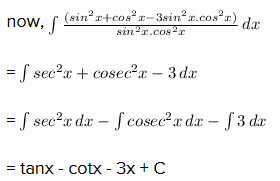# Integrate the following:-∫(sin^6 x+cos^6 x)/sin^2 x.cos^2 x dx

5 years ago

First note that sin6(x)+cos6(x) is a sum of two cubes (sin2(x))3+(cos2(x))3 so it can be factored using a3+b3=(a+b)(a2ab+b2) and the Pythagorean identity to get

sin6(x)+cos6(x)=(sin2(x))3+(cos2(x))3

=(sin2(x)+cos2(x))(sin4(x)sin2(x)cos2(x)+cos4(x))

=sin4(x)sin2(x)cos2(x)+cos4(x).

Therefore,

sin6x+cos6xsin2(x)cos2(x)=sin4(x)sin2(x)cos2(x)+cos4(x)sin2(x)cos2(x)

=tan2(x)1+cot2(x)

But tan2(x)=sec2(x)1 and cot2(x)=csc2(x)1. Hence,

sin6x+cos6xsin2(x)cos2(x)=sec2(x)+csc2(x)3 and thus

sin6(x)+cos6(x)sin2(x)cos2(x) dx=sec2(x)+csc2(x)3 dx

=tan(x)cot(x)3x+C

3 years ago
=sin6(x)/sin2x.cos2x+cos6(x)/sin2x.cos2x
=sin4x/cos2x+cos4x/sin2x
=(1-cos2x)2/cos2x + (1-sin2x)2/sin2x
=(1-2cos2x+cos4x)/cos2x + (1-2sin2x+sin4x)/sin2x
=sec2x -2 + cos2x + cosec2x -2 + sin2x
=sec2x + cosec2x -4 + 1
=sec2x + cosec2x -3
so, by integration we get,
=tan x – cot x -3x + C

2 years ago
Dear Student,